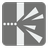Shooting Range
Simple
English RU

On a shooting range, shooters use a wall to practice, trying to hit it from various positions. Shooters do not have problems with with vertical deviation, so we can use a simplified model for this mission. We placed a camera above the shooting range and use Cartesian coordinates to describe states. We know coordinates of wall (target) ends and shooting point. And we know the point where is a bullet after a small time. You should determine the result of the shot.

You are given coordinates for the end-points of the target wall on a grid. In addition, you have two points describing the shot: A starting point where the bullet was fired, and....Four arguments. Two wall ends, a firing point and a later point as tuples of two numbers.

The results as an integer from -1 to 100.

```shot((2, 2), (5, 7), (11, 2), (8, 3)) == 100
shot((2, 2), (5, 7), (11, 2), (7, 2)) == 0
shot((2, 2), (5, 7), (11, 2), (8, 4)) == 29
shot((2, 2), (5, 7), (11, 2), (9, 5)) == -1
shot((2, 2), (5, 7), (11, 2), (10.5, 3)) == -1
```

This concept can be using for modeling software and could help to determine the visibility of an object from a particular vantage point, or to calculate the trajectory of an object. For example, the trajectory of the ball in Pong, or a missile in Missile Command.

all(all(0 < i < 100 for i in coor) for coor in args)
wall1, wall2 and shot_point are not collinear.

You should be an authorized user in order to see the full description and start solving this mission.